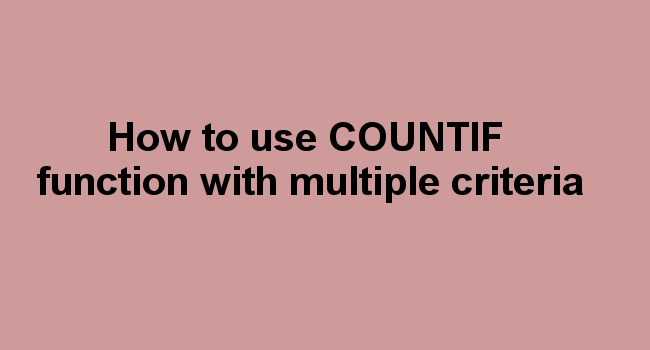## How to use COUNTIF function with multiple criteria

• Post author:
• Post category:Formula

COUNTIF function is one of the most common excel function used for counting numbers within a specific range. The number of cells in the range should match the supplied criteria.

Users need to specify a single or multiple criteria to get the result. If you want to count cells based on multiple criteria, you can use a combination of COUNTIF functions or use COUNTIFS function. This tutorial will teach you how to use countif or countifs for single and multiple conditions.

COUNTIF function returns a numeric value and be applied to Microsoft Excel 2016, Excel 2013, Excel 2011 for Mac, Excel 2010, Excel 2007, Excel 2003 and Excel 2000.

Syntax: single criteria

COUNTIF (range, Criteria)

COUNTIF Parameters or Arguments

1. Range: This is a range of cells to be counted based on the criteria.

2. Criteria: It is the criteria used to determine which cells to be counted.

Example 1: From the table below, Count the number of cells with Bananas.Put your cursor on cell D5 and click on FX to insert function.Enter function argumentsClick OK.=COUNTIF (A2:A7,A5) – Counts the number of cells with Bananas from A2 to A7 and returns 1.

Example 2:

Count the number of cells with a value greater than 2 in cells C2 through to C7The result is 2

## Count cells with multiple criteria

### A. Using COUNTIF function for Multiple Criteria

COUNTIF can be used to count two or more criteria by adding or subtracting several COUNTIF functions.

Syntax

=COUNTIF (range, Criteria)+ COUNTIF (range, Criteria)+..

Parameters

1. Range: This is a range of cells to be counted based on the criteria.

2. Criteria: It is the criteria used to determine which cells to be counted.

Example 1: COUNTIF with two conditionsClick OK=COUNTIF(A2:A9,A4) + COUNTIF(A2:A9,A2)

The formula counts the number of apples (the value in cell A4) and lemons (cell A2) in cells A2 through to cell A9. The COUNTIF formula is used twice to specify multiple criteria.

### B. Using COUNTIFS function for multiple criteria

COUNTIFS Function can also be used to specify multiple criteria. The "s" makes it plural. The function works the same as COUNTIF except that, the added criteria is separated by commas.

Syntax

=COUNTIFS (Criteria_range1, Criteria1, [Criteria_range2, Criteria2] …)

Parameters or arguments

1. Criteria_range1: The range of cells for which you want to evaluate against Criteria 1.

2. Criteria 1: it is the criteria used to evaluate for Criteria_range1 to determine the cells to count.

3. [criteria_range2]: is the range of cells you use to evaluate against criteria 2.

4. [Criteria]: Is the criteria which you want to evaluate for Criteria_range2 to determine which cells to count.After entering the range of cells press f4 key on the keyboard to lock the cells. Then press OK.